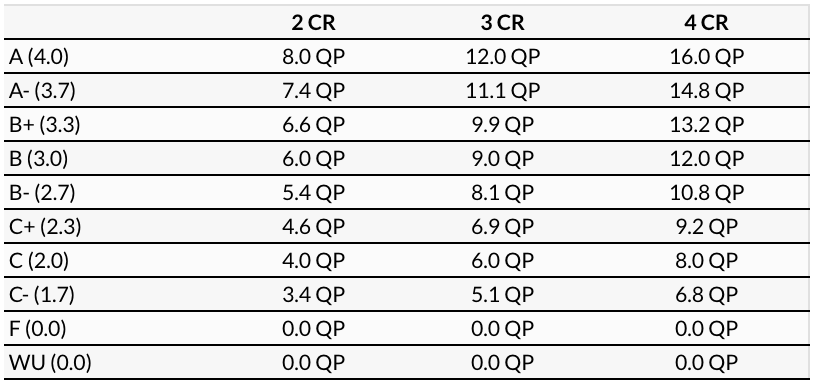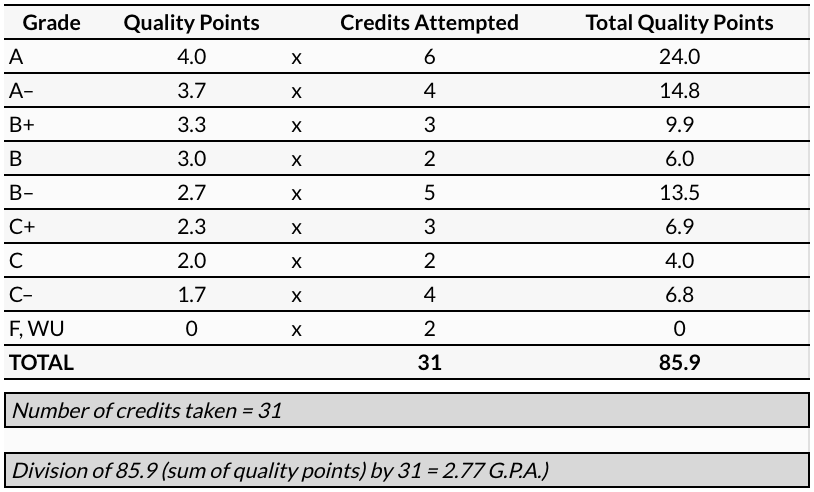# Grade Point Average (GPA) Computation Formula

Quality points (QP) are calculated by multiplying the credit value of each course by the numerical value of the grade received, 4.0 to 1.7 for grades A through C-, and 0 for F or WU. (See the following table.)• Add the number of credits taken, including those with F or WU grades.

• Divide the total number of quality points by the total number of credits attempted. This final figure is the GPA.

Both matriculated and nonmatriculated graduate students must maintain a minimum GPA of 3.0 to remain in good standing at Lehman College. Matriculants require a minimum GPA of 3.0 for graduation. Undergraduate-level courses and courses transferred from other institutions are not included in computing the GPA.

### Sample G.P.A. Computation250 Bedford Park Boulevard West
Bronx, NY 10468 | 718-960-8000
Policies